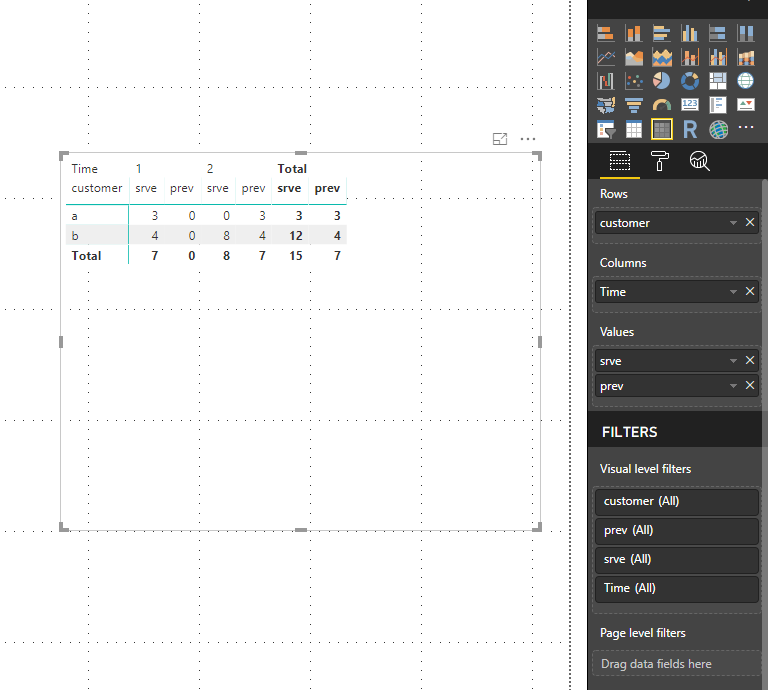cancel
Showing results for
Did you mean:Helper II

## Window functions which I never got to work in Dax

I have a table here:I'd like to make power pivot that looks at the value at a prior time even if there isn't a value in the current time

I used:

srev = sum(value)

prev=CALCULATE([srev],filter(all(Table1[time]),Table1[time]=max(Table1[time])-1))

And got:b.prev at 2 = 4 which is the same as srev at 1.

a.prev at 2 is blank because there is no row at 2.  But there is a prev value at 2.  It should be 3.  So what's the right equation.

Also I'd like another equation that equals 1 when prev is 1 and srev = 0 grouped by company.  Then the grand total should be = 1 in time 2 because a should have been 1 in time 2 and b should be 0.  I did:

This didn't work because the addcolumns thingy showed blanks for both srev and prev.

2 REPLIES 2Microsoft

@Ffitzpatrick47,

For PowerPivot solutions, please post the question in PowerPivot forum to get dedicated support.

For Power BI solutions, please firstly create a Time table as below. Create relationship between Time table and your original table.Then create the following measure in your original table.

`srve = SUM(Table1[value])+0`
`prev = CALCULATE([srve],filter(ALL('Time'),'Time'[Time]=max('Time'[Time])-1))`

At last, create a Matrix visual as follows, please note that , it is not possible to put measures into Rows of Matrix.For your second requirement, please check if measure and measure2 in attched PBIX file return the correct result, if not, please post expected result based on the sample data.

Regards,
Lydia

Community Support Team _ Lydia Zhang
If this post helps, then please consider Accept it as the solution to help the other members find it more quickly.Helper II

I should mention a solution like this:

Wouldn't work because I actually do need to know the difference between a blank and a 0 in real life.